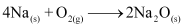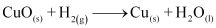### Chemical Reaction and Equation Chapter Wise Important Questions Class 10 Science

#### This are Important Questions of Chapter 1 Science Chemical Reaction and  Equation will help you in scoring more marks.

1.we need to balance a skeletal chemical equation Give reason to justify the statement.

2.Consider the following chemical reaction.

X+Barium chloride → Y Sodium chloride.
(White ppt)

(a) Identify "X'' and  ''Y''
(b) The type of reaction.

3.(i) Write a balanced chemical equation for process of photosynthesis.
(ii)When do desert plants take up carbon dioxide and perform photosynthesis?

4.Write the chemical equation of the reaction in which the following changes have taken place with an example of each:

(i) Change in colour.
(ii) Change in temperature.
(iii) Formation of precipitate.

5.State the type of chemical reactions and chemical equations that take place in the following:
(i) Magnesium wire is burnt in air.
(ii) Electric current is passed through water.
(iii) Ammonia and hydrogen chloride gases'are mixed.

6. Define a balanced chemical equation. Why should an equation be balanced? Write the balanced chemical equation for the following reaction:
(i) Burning of natural gas
(ii) The process of respiration

7.A solution of a substance ‘X’ is used for white washing.
(i) Name the substance ‘X’ and write its formula.
(ii) Write the reaction of the substance ‘X’ named in (i) above with water.

8.Identify the substances that are oxidised and the substances that are reduced in the
following reactions.

(i)(ii)9. What is rancidity ? Mention any two way by which rancidity can prevented.

10.State one basic difference between a physical and chemical change.

1.